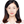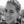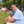## CORRECTION article

Front. Integr. Neurosci., 17 September 2021
https://doi.org/10.3389/fnint.2021.745196

# Corrigendum: Estimating the Minimal Number of Repeated Examinations for Random Responsiveness With the Coma Recovery Scale—Revised as an ExampleHao Yang1,Chengyin Ye2,Xiaochen Liu1,Lingxiu Sun1,Anqi Wang1,Jing Wang1,Nantu Hu1,Xiaohua Hu3,Olivia Gosseries4,Steven Laureys4,Haibo Di1* andJiqian Fang5*
• 1International Unresponsive Wakefulness Syndrome and Consciousness Science Institute, Hangzhou Normal University, Hangzhou, China
• 2Department of Health Management, School of Public Health, Hangzhou Normal University, Hangzhou, China
• 3Hangzhou Wujing Hospital, Hangzhou, China
• 4Coma Science Group, GIGA- Consciousness and Centre du Cerveau, University and University Hospital of Liège, Liège, Belgium
• 5School of Public Health, Sun Yat-sen University, Guangzhou, China

by Yang, H., Ye, C., Liu, X., Sun, L., Wang, A., Wang, J., Hu, N., Hu, X., Gosseries, O., Laureys, S., Di, H. and Fang, J. ( (2021). Front. Integr. Neurosci. 15:685627. doi: 10.3389/fnint.2021.685627

In the original article, there was a mistake in the legend for Table 5 as published. “${\stackrel{^}{k}}_{min}$” was missed. The correct legend appears below.

The numbers of repeated examination ${\stackrel{^}{k}}_{min}$ for pip, p~N(p, 0.32) and pi~U(p−0.3,p+0.3) *

In the original article, there was a mistake in Table 1 as published. The mathematical symbols were misexpressed. The corrected Table 1 appears below.

TABLE 1

Table 1. Pooled estimate for the probability of positive response to a single examination given by an MCS patient in theory.

In the original article, there was a mistake in Table 2 as published. The mathematical symbols were misexpressed. The corrected Table 2 appears below.

TABLE 2

Table 2. Estimation for a total number of MCS patients and their probability of giving positive response to a single examination.

In the original article, there was a mistake in Table 3 as published. The mathematical symbols were misexpressed. The corrected Table 3 appears below.

TABLE 3

Table 3. The data collected from the 13 rounds of successive examinations.

In the original article, there was some errors. The mathematical symbols were misexpressed. A correction has been made to Materials and methods, Development of statistical formulas, Data and formulas, Paragraph 2:

Since both of a1/n and any of the formulas in the 5-th column of Table 1 approximate to the same probability of positive response to a single examination given by a MCS patient, we have

$a1n≈a1+a2n+(n-a1),a1n≈a1+a2+a3n+(n-a1)+(n-a1-a2)$

and

$a1n≈a1+⋯+ain+(n-a1)+⋯(n-a1-⋯-ai-1)$

Denote their solutions of n, respectively, by

and

These formulas have been summarized in Table 2.

A correction has been made to Materials and methods, Validation by stochastic simulation, “Examination” and “responses,” Paragraph 1: [0, 1]. Paragraph 3: $\stackrel{^}{n}=\sum _{i=1}^{{\stackrel{^}{k}}_{min}}{a}_{i}$, the rate of missed diagnosis $\left(n-\stackrel{^}{n}\right)/n$.

A correction has been made to Materials and methods, Validation by stochastic simulation, Repeated simulation and the rate of missed diagnosis, Paragraph 1: $\left(n-\stackrel{^}{n}\right)/n$.

A correction has been made to Results, Outcome of Bedside examinations, For TBI patients, Paragraph 1–6:

After completing the first 2 rounds of examinations we obtained the numbers of MCSs giving positive response a1 = 30 and a2 = 3, using the formulas in the second row of Table 2, we had the estimated n and p as

$n^2≈a12a1-a2=90027=33.33,p^2≈a1n^2=3033.33=0.9001$

Since

$(1-p^2)k≥0.0001,k=2,3,4,(1-p^2)5=0.00001<0.0001$

the examination should be kept on going, and might be ended at the 5-th round; and the total number of MCS patients in this group of DOCs might be around 34.

After completing the 3rd round, we obtained a3 = 3, and

$n^3≈a1(2a1+a2)2a1-a2-a3=30×6354=35,p^3≈a1n^3=3035=0.8571$

Since

the examination should be kept on going, and might be ended at the 5-th round; and the total number of MCS patients in this group of DOCs might be around 35.

After completing the 4-th round, we obtained a4 = 2, and

$n^4≈a1(3a1+2a2+a3)3a1-a2-a3-a4=30×9982=36.22,p^4≈a1n^4=3036.22=0.8283$

Since

$(1-p^4)i≥0.0001,i=4,5,(1-p^4)6=0.00003<0.0001$

the examination should be kept on going, and might be ended at the 6-th round, and the total number of MCS patients in this group of DOCs might be around 37.

After completing the 5-th round, we obtained a5 = 0, and

Since

$(1-p^5)5=0.00020,(1-p^5)6=0.00004<0.0001$

the examination should be kept on going, and perhaps ended at the 6-th round; and the total number of MCS patients in this group of DOCs might be around 37.

After completing the 6-th round, we obtained a6 = 0, and

Since

the examination could be ended at this round ${\stackrel{^}{k}}_{\text{min}}=6$.

And up to this round, the total number of MCS patients had been detected in the group of DOCs was

$n^=a1+a2+a3+a4+a5+a6=38$

A correction has been made to Results, Outcome of Bedside examinations, For NTBI patients, Paragraph 1–4:

After completing the first two rounds of examination we obtained the numbers of MCSs giving positive response a1 = 29 and a2 = 3, using the formulas in the second row of Table 2, we had

$n^2=a12a1-a2=29226=32.35,p^2≈a1n^2=2932.35=0.8966$

Since

the examination should be kept on going, and might be ended at the 5-th round; and the total number of MCS patients in this group of DOCs might be 33.

After completing the 3rd round, we obtained a3 = 2, and

Since

the examination should be kept on going, and might be ended at the 5-th round; and the total number of MCS patients in this group of DOCs might be 34.

After completing the 4-th round, we obtained a4 = 1, and

$n^4=a1(3a1+2a2+a3)3a1-a2-a3-a4=29×9581=34.01,p^4≈a1n^4=2934.01=0.8526$

Since

the examination could be ended at 5-th round; and the total number of MCS patients in this group of DOCs might be 35.

After completing the 5-th round, we obtained a5 = 0, and

$n^5=a1(4a1+3a2+2a3+a4)4a1-a2-a3-a4-a5=29×130110=34.27,p^5≈a1n^5=2934.27=0.8462$

Since

$(1-p^5)5=0.000086<0.0001$

the examination could be ended at this round ${\stackrel{^}{k}}_{\text{min}}=5$ and up to this round, the total number of MCS patients had been detected in the group of DOCs was

$n^=a1+a2+a3+a4+a5=35$

The authors apologize for the errors and state that this does not change the scientific conclusions of the article in any way. The original article has been updated.

## Publisher's Note

All claims expressed in this article are solely those of the authors and do not necessarily represent those of their affiliated organizations, or those of the publisher, the editors and the reviewers. Any product that may be evaluated in this article, or claim that may be made by its manufacturer, is not guaranteed or endorsed by the publisher.

Keywords: repeated examinations, random responsiveness, diagnosis, Coma Recovery Scale-Revised, disorders of consciousness, minimally conscious state

Citation: Yang H, Ye C, Liu X, Sun L, Wang A, Wang J, Hu N, Hu X, Gosseries O, Laureys S, Di H and Fang J (2021) Corrigendum: Estimating the Minimal Number of Repeated Examinations for Random Responsiveness With the Coma Recovery Scale—Revised as an Example. Front. Integr. Neurosci. 15:745196. doi: 10.3389/fnint.2021.745196

Received: 21 July 2021; Accepted: 03 August 2021;
Published: 17 September 2021.

Edited by:

Ewelina Anna Knapska, Nencki Institute of Experimental Biology (PAS), Poland

Reviewed by:

Piotr Jerzy Durka, University of Warsaw, Poland

Copyright © 2021 Yang, Ye, Liu, Sun, Wang, Wang, Hu, Hu, Gosseries, Laureys, Di and Fang. This is an open-access article distributed under the terms of the Creative Commons Attribution License (CC BY). The use, distribution or reproduction in other forums is permitted, provided the original author(s) and the copyright owner(s) are credited and that the original publication in this journal is cited, in accordance with accepted academic practice. No use, distribution or reproduction is permitted which does not comply with these terms.

*Correspondence: Jiqian Fang, fangjq@mail.sysu.edu.cn; Haibo Di, dihaibo19@aliyun.com

These authors have contributed equally to this work and share first authorship